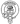# Introduction to ES Modules

June 25, 2020

The ECMAScript Modules system brings an official, standardised module system to JavaScript. While Node.js has been using the CommonJS standard for years now there wasn’t an official standard for use in the browser. Modules give you a better way to organize your variables and functions, allowing you to split up your code and share functionality through explicit relationships.

# Syntax

Before we deep-dive the syntax we first need to define what a module is in JavaScript:

A module is a JavaScript file that exports one or more values (objects, functions or variables), using the export keyword.

In order to import one of these modules we use the `import` keyword. Let’s take a look at a simple example:

``import package from 'module-name'``

And to export something from a module we use the `export` keyword like so:

``````// add.js

export default (x, y) => x + y``````

This is just a simple function that takes two numbers, adds them together and returns the result. So what’s with the `default` in there? Well by defining a single default export we can keep it as an anonymous function. If we wanted to export other things from this module we would need to give it a name.

Here’s how we would import and use our shiny new add module:

``````import add from './add.js'

// This add module can actually also be used to concatenate strings
add('Hello ', 'World') // returns Hello World``````

Because our module is a default export we actually don’t give it a name until we import it so we could switch out add for whatever we prefer here:

``````import veryVeryCoolAdd from './add.js'

# Multiple Exports

As mentioned above we can’t use the above syntax to export more than one thing so we’ll need to make some changes.

``````// math.js

const add = (x, y) => x + y
const subtract = (x, y) => x - y
const multiply = (x, y) => x * y
const divide = (x, y) => x / y

export { add, subtract, multiply, divide }``````

Then we can import everything from this module using the * character.

``````import * from './math.js'

subtract(2,1) // returns 1
multiply(3,3) // returns 9
divide(4,2) // returns 2``````

Or by leveraging destructuring we can import only a few of the exports.

``````import { add, subtract } from './math.js'

subtract(2, 1) // returns 1``````

You can even rename any of your imports, using as:

``````import { add, subtract as veryVeryCoolSubtract } from './math.js'

veryVeryCoolSubtract(2, 1) // returns 1``````

It’s even possible to import a default export in combination with a named export.

``````// math.js

export default (x, y) => x + y
const subtract = (x, y) => x - y
const multiply = (x, y) => x * y
const divide = (x, y) => x / y

export { subtract, multiply, divide }``````
``````import add, { subtract, multiply, divide } from './math.js'